# Hydrostatic Blues

## March 8, 2017

In the last post, I described the analytic calculation I’m doing in order to set up a 3D disk in vertical hydrostatic equilibrium with my NFW + Miyamoto-Nagai profile. Here’s a density projection of what the resulting disk looks like: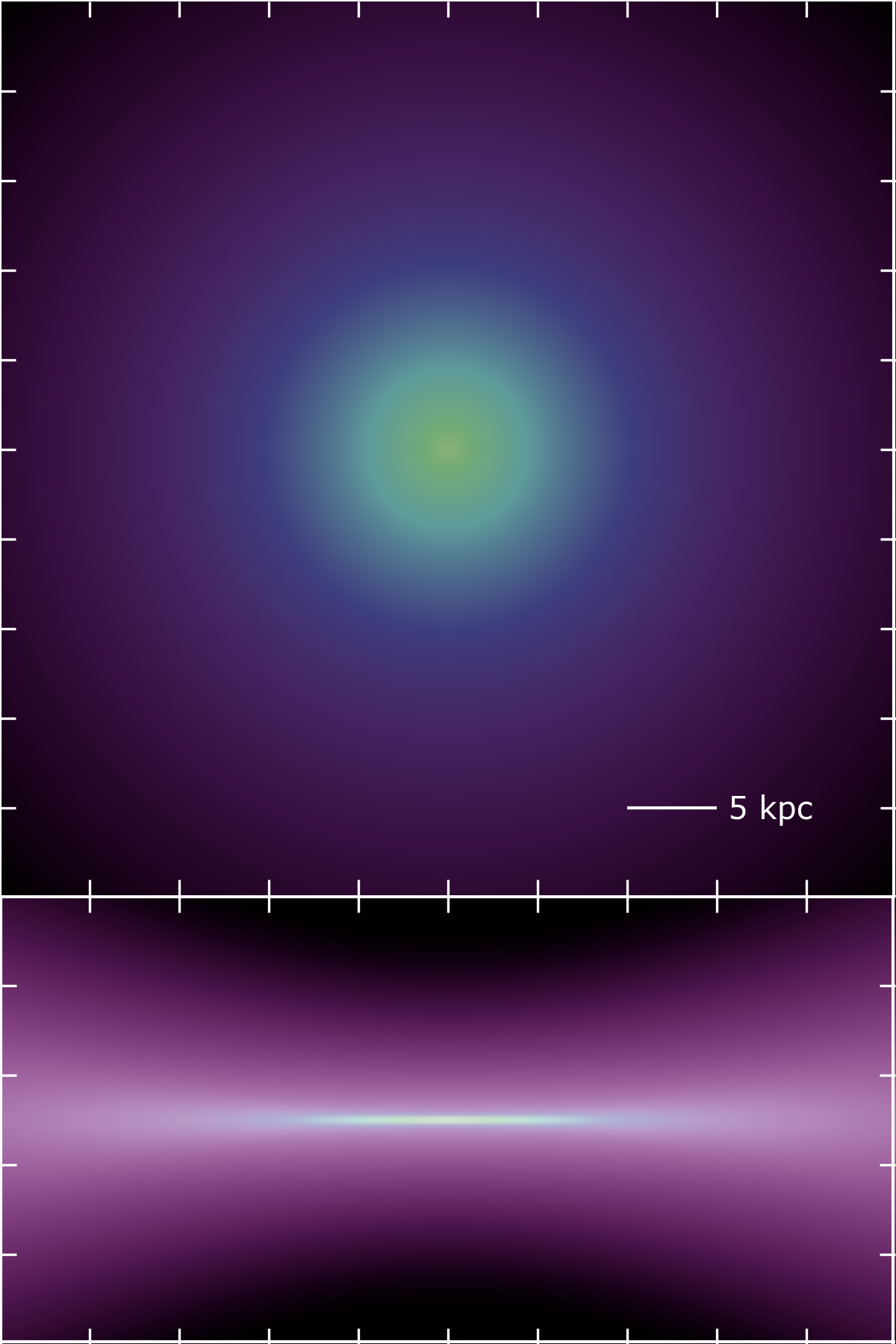Pretty, right? Unfortunately, when I try to evolve this disk, things break pretty quickly. This post is dedicated to figuring out why.

First, I had to confirm that the hydrostatic calculation was the source of my problems. To check this, I ran the simulation with vertical gravity turned off. As expected, the disk evolves just fine in this scenario, puffing up vertically as a result of the pressure gradient I’ve implemented. Here’s picture after a little time has passed: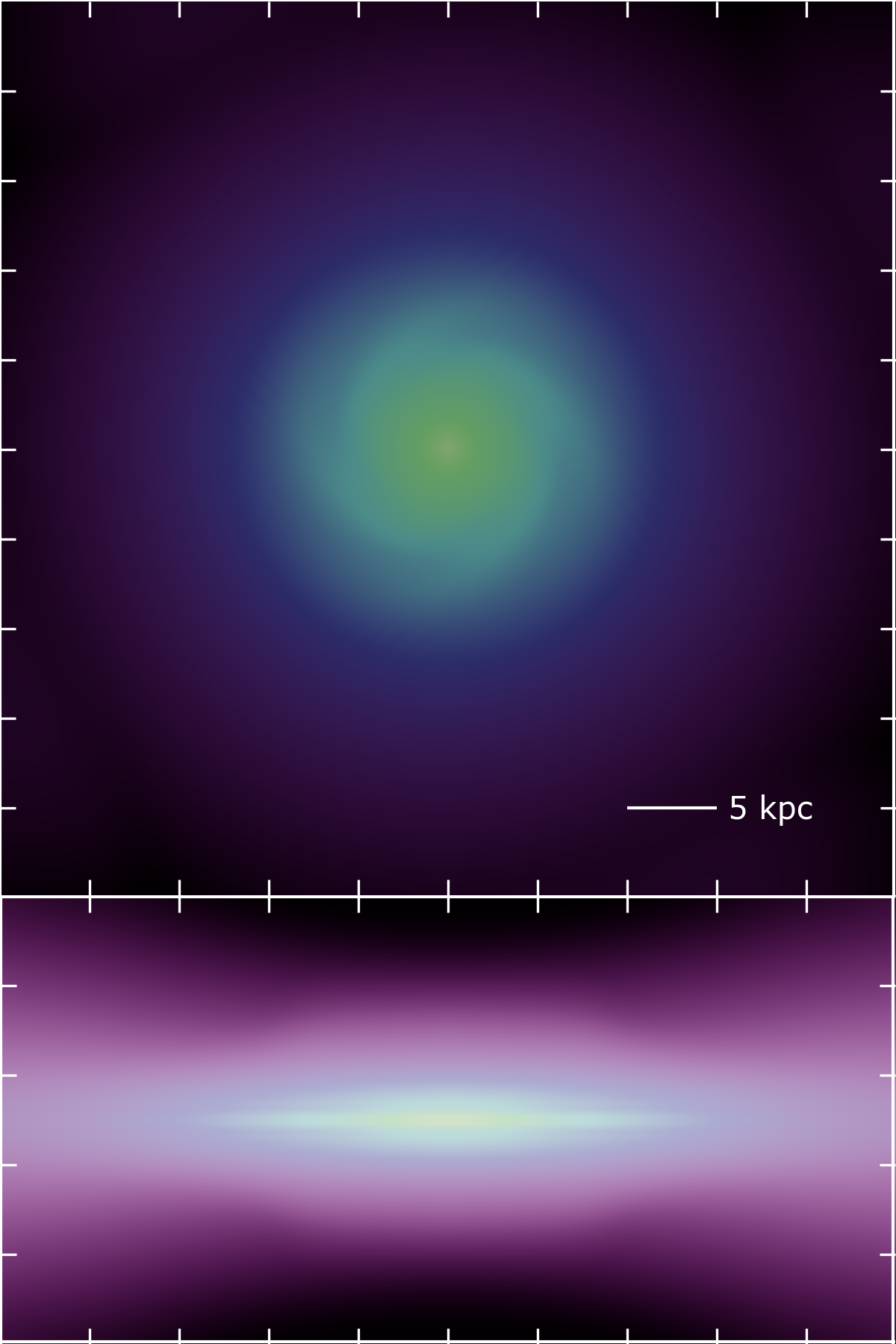As soon as I turn on gravity, the simulation goes haywire. Relatively quickly, cells near the center of the disk wind up with negative densities. Given that the central radii are the locations with the steepest density and pressure gradients, this is not altogether surprising.

So, assuming the problem is the failure of hydrostatic balance, what could be causing it? Well, first, the analytic calculation of the pressure gradient could be wrong, or the numerical implementation of that pressure gradient could be wrong. To rule these out, I’ve done a 1D simulation of a single column in z at a radius far enough out in the disk that the pressure gradient is well resolved. Here’s how the density evolves in that case:

Not perfect, but it doesn’t break, and gets close to achieving hydrostatic balance. Certainly the regions near $z = 0$ look pretty good. The trouble here seems to be the outer regions, where I’ve arbitrarily set a density floor of 1 ($n_h \approx 10^{-6}\mathrm{cm}^{-3}$). The pressure gradient in the outer regions is 0, but there’s still a force due to gravity that is proportional to the density.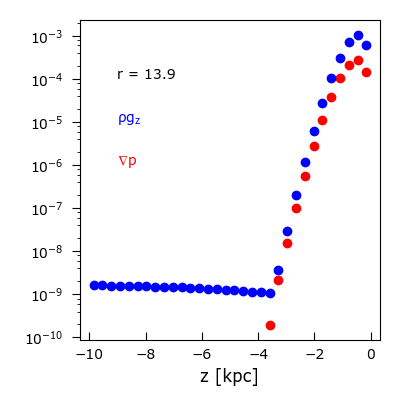Over time, the lack of balance in the transition region propagates further in, causing the density of the outer cells to drop, and the density of the inner cells to increase. In this case, the densities are low enough that the net effect on the inner regions is small, and the column stays mostly balanced.

In regions at smaller radii, where the pressure gradient is less well resolved, the problem gets much more severe. Below are two movies showing the evolution of the density in a column with a radius of $r = 6.9$kpc, and one at the innermost radius in this simulation, $r = 0.2$kpc.

These don’t run as long, because in both cases the code grinds to a halt just after the movie ends. Clearly, this is a problem that could cause the code to break when I evolve the disk in 3D. To better understand exactly what’s upsetting the balance, I again plot the pressure gradient and compare it to $\rho g_{z}$ for the $r = 6.9$kpc case: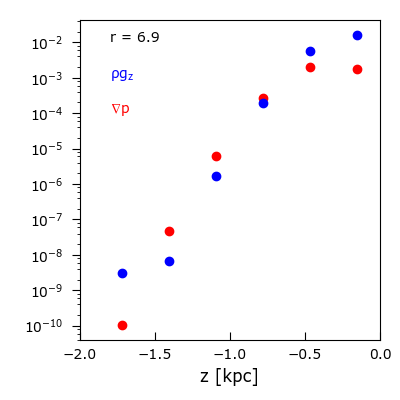Here, I’ve blown up the region with all the action - just outside $z = 2$ the plot looks identical to the $r = 13$kpc case. In all the plots above, there are only 62 cells in the $z$-direction. What happens if I increase the resolution? Well, the code still crunches to a halt. Here’s what the pressure balance plots look like: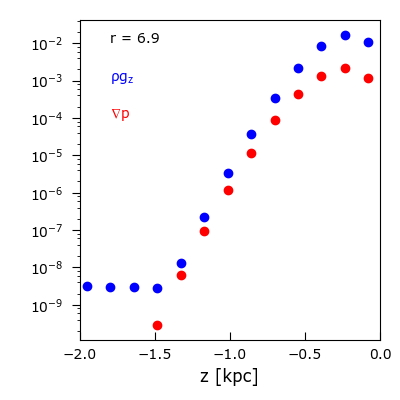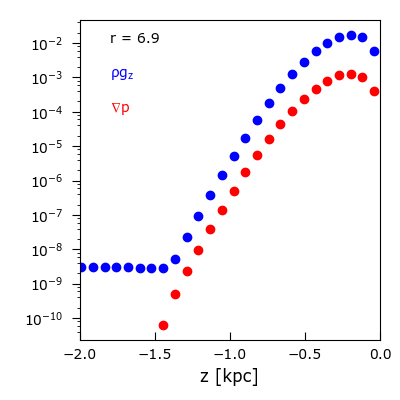Evidently, better resolving the gradient in $z$ doesn’t fix the problem. In fact, it seems to be making it worse.